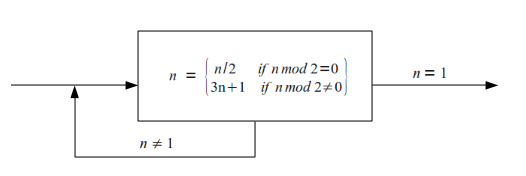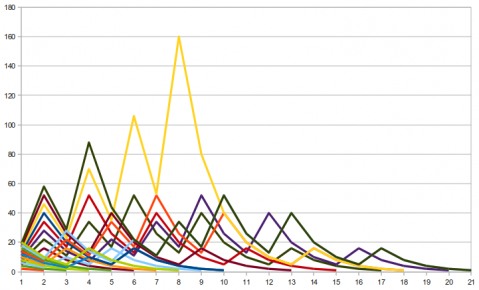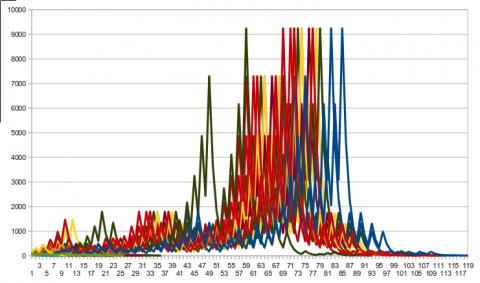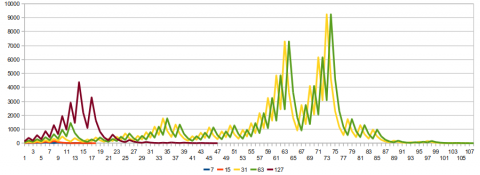Global Constant
Steve Nay's ramblings

# Some notes on the Collatz Conjecture

Part of my Computational Theory (CS 252) class this semester deals with the proof (or rather the lack thereof) of the Collatz conjecture. We were assigned a group problem to “prove” the conjecture, the point being, of course, that we would examine it and try to find patterns (since the likelihood of a few undergraduate CS students discovering such a proof is rather small). I found the conjecture fascinating and decided to post a few of the observations made by our group and myself.

Here is a flowchart of how the Collatz conjecture works:One of our group members wrote a short Java program to run the Collatz conjecture on arbitrary input. I ran it on two data sets, one with the natural numbers {1..20} and one with the natural numbers {1..100}. I imported these into a spreadsheet and generated graphs of each of them. Here’s the graph over the {1..20} set:• {15} maxed out at 160 (max of the whole range)
• {19} maxed out at 88
• {7, 9, 11, 14, 17, 18} maxed out at 52
• All of these integers reduce to 17 somewhere along the line, and 52 is the next iteration after 17

It’s also interesting to note that the even integers {1..20} start going down first on the graph, and the odd numbers go up first. The odd integers usually, but not always, max out higher than the even integers.

This is the graph of the set of integers {1..100}:Of the integers in this set, {27, 31, 41, 47, 54, 55, 62, 63, 71, 73, 82, 83, 91, 94, 95, 97} max out at 9232 (which is the max of the entire range).

Another of our group members noticed an interesting pattern in the output for the integers in the set {7, 15, 32, 63}. These integers are related to the number preceding them in the set by the formula 3n + 1. For example, 3 * 7 + 1 = 15. Here is the sequence generated by Collatz for 7:

(7, 22, 11, 34, 17, 52, 26, 13, 40, 20, 10, 5, 16, 8, 4, 2, 1)

And for 15:

(15, 46, 23, 70, 35, 106, 53, 160, 80, 40, 20, 10, 5, 16, 8, 4, 2, 1)

First of all, you can note that the last 9 elements of both of these sequences are the same. That is, once 7 is reduced to 13, the next element in the sequence is 40, and once 15 is reduced to 80, the next element in the sequence is also 40. Once the two have reached that common point, the rest of their sequences are the same.

But the intriguing observation is the bolded numbers in each sequence. in the 15 sequence, we eventually reach 35, which is one greater than 34 in the 7 sequence. Each sequence then has some arbitrary number, after which the next element of each sequence again differs by one. In the case of 7 and 15, this occurs only twice. The same pattern can be seen in the sequences for 31 and 63, but they are not reproduced here. (See the CSV files below for the data.)

After 63, however, the pattern breaks. 127 does not exhibit this phenomenon. A graph of the sequences for {7, 15, 31, 63, 127} shows this:Here is apparent that 127 reduces fairly quickly, compared to 31 and 63, for example. This shows that 127 fails to carry on the pattern observed for the integers {7, 15, 31, 63}.

In step 13 of the 31 sequence and step 14 of the 63 sequence, both sequences reduce to 364. After this point, they parallel each other (differing by one step) until they reduce to 1. This is easy to spot in the above graph, and it helps explain the clusters of similarly shaped lines for the sequences in, for example, the graph over the integers {1..100}.

The data used for these graphs can be downloaded here ({1..20}), here ({1..100}), and here ({7, 15, 31, 63, 127}). The data series are on the rows and are comma-separated.